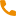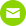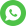la langue+86(0086) 18130250095[email protected]
categories
récent des postes
Mots clés
Calculation formula of pump lift

2018/10/11

The centrifugal chemical pump with specific speed ns of 130 and 150 is usually selected. The flow rate of the electric chemical pump should be 1.1 and 1.2 times of the rated flow of the chillers (1.1 for a single pump and 1.2 for two parallel pumps).The estimated loss per 100m pipe length is 5mH2O, pump head (mH2O):

Hmax = P1 + P2 + 0.05L (1 + K).

P1 is the water pressure drop of the evaporator of the chiller.

P2 is the water pressure drop of the unit with the largest water pressure loss of each unit in the parallel connection of the ring.

L shall be the length of the least favourable loop.

K is the ratio of the sum of equivalent length of local resistance in the most unfavorable loop to the total length of the straight pipe. When the most unfavorable loop is longer, the value of K is 0.2 ~ 0.3, and when the worst loop is shorter, the value of K is 0.4,0.6.

Methodology 2:

What we are talking about here is the drag component of a closed air conditioning cold water system, because this is the most commonly used system.

1. Chillers resistance: provided by the unit manufacturer, generally 60~100kPa.

2. Pipe resistance: including friction resistance, local resistance, where the unit length of friction resistance than friction resistance depends on technical and economic comparison.If the value is large, the pipe diameter is small, and the initial investment is small, but the energy consumption of the pump is large; if the value is small, the reverse is true.At present, the design of cold water pipeline than the group should be controlled in the 150~200Pa/m range, pipe diameter larger, the value may be smaller.

3. Air conditioning not end device resistance: the end device type has the fan coil unit, the combination type air conditioner and so on.Their resistance is based on the design of the air in and out of the air conditioning coil parameters, cooling capacity, water temperature difference by the manufacturer after the calculation of coil configuration, many rated working conditions can be found on the product samples.This resistance is generally within the 20~50kPa range.

4. Control valve resistance: air conditioning room temperature is always required to control, through the installation of water at the end of the air conditioning device electric two-way control valve is a means to achieve room temperature control.The size of the two-way valve is selected by the flow capacity and the allowable pressure drop when the valve is fully open.If the value of the allowable pressure drop is large, the control performance of the valve is good; if the value is small, the control performance is poor.The percentage of the pressure drop in the total pressure drop of the branch when the valve is fully open is referred to as the valve weight.The design of the water system requires a valve weight of S & GT, 0.3, so the allowable pressure drop of the two-way control valve is generally not less than that of the 40kPa.

Based on the above, the pressure loss of the air conditioning water system in a tall building of about 100 m high can be roughly estimated, that is, the lift required for the Chemical circulating pump:

1. Resistance of chillers: 80 kPa (8m water column);

2. Pipe resistance: take the resistance of defiler, water collector, water divider and pipeline in the freezer room to be 50 KPA; take the pipeline length of transmission side 300 m and specific friction resistance 200 Pa / m, the friction resistance is 300, 200 and 60 000 Pa=60 KPA; if the local resistance of transmission and distribution side is taken into account, the friction resistance will be 300 KPA, 200 KPA and 60 000 KPA.When the friction force is 50% of the friction resistance, the local resistance is 60 kPa*0.5=30 KPA, the total resistance of the system pipeline is 50 kPa+60 kPa+30 kPa=140 kPa (14 m water column);

3. Air conditioning end equipment resistance: the resistance of the combined air conditioner is generally larger than that of the fan-coil unit, so the resistance of the former is 45 kPa (4. 5 water column).

4. Two-way control valve resistance: 40 kPa (4m water column).

5. So, the sum of the parts of the water system is: 80 kPa+140kPa+45 kPa+40 kPa=305 kPa (30. 5 m water column).

6. Pump lift: take 10% of the safety factor, head H=30.5m*1.1=33.55m.

According to the above estimated results, the range of the pressure loss value of the air conditioning water system of the same scale buildings can be basically grasped, and the overestimation of the pressure loss of the system should be prevented because it is too conservative and has not been calculated.Pump head chosen too large, resulting in a waste of energy.

Related reading:Magnetic Chemical Pump, Electric Diaphragm Pump

l\'équipe professionnelle au service !

•+86(0086) 18130250095
•+86+0563-5093319
•[email protected]
•live:f39b9a5d3fd4ef7
•+8619956347488